www.batmath.it

### Galilean relativity and Galileo's transformations

The principle of Galilean relativity asserts that all the inertial systems of reference are physically equivalent: no experiment performed inside a given system of reference can highlight the rectilinear and uniform motion of the same system, or, in other words, the physical laws found by experimenters who work in laboratories in relative rectilinear and uniform motion must have the same shape. Now we must deduce the formulas that link the time-space coordinates of an event seen from two different observers and show that the laws of physics are invariant in the passage from one frame of reference to another (in shape): in short we must translate into formulas the content of this principle.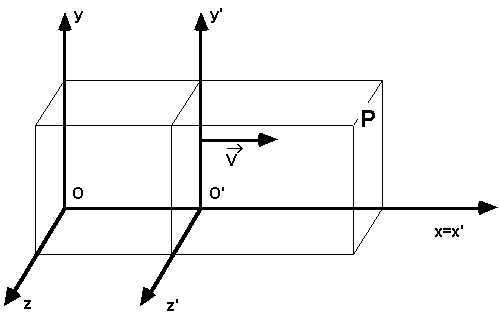Let us consider two frames of reference, S and S’. We suppose that S’is moving, with respect to S, in a rectilinear and uniform motion, with speed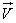. Let us suppose that the observers S and S’ have two clocks to measure times, previously synchronised in such a way,  for example, that, when O is upon O’, both clocks point to zero.

It is not limiting to suppose, as we will do, the x axes of the two systems of reference one upon the other, so as to haveparallel to the same x axis. Let us consider a certain physical event that happens in a point P with co-ordinates (x, y, z) and (x’, y’, z’) with respect to S and S’ and at times t and t’ measured by the two observers.

Remembering that OO’ = vt and that it seems obvious to suppose t=t’, from the picture by side it follows immediately the validity of the:

Galilean Transformations: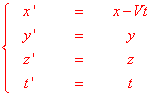We observe in particular that the fourth of these formulas has always been considered as obvious and it still is in everyday life. The refusal of the hypothesis (because this is an hypothesis and not a law proved by way of experiments) that the watches of the two observers should mark the same time is, as we will see, one of the innovative aspects of Einstein relativity.

With a first differentiation we can obtain the formulas for the composition of speeds: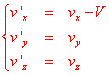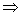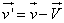With another differentiation we can arrive to the fundamental conclusion that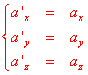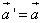If we consider the fact that the mass of bodies is an unchangeable characteristic, using the definition of force, that is the principal equation of dynamics,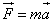, we will conclude that the form of the equations is indipendent from the reference system. If we want to preserve the principle of relativity, an alteration to Galileo's transformations must give rise to a necessary modification of the principle of dynamics. This change in the fundamental law of dynamics is one of the main outcomes of the theory of relativity. It's important to point out that Einstein's special theory of relaivity has not denied the validity of Galileo's relativity, but only of the mathematical formulas used in the passage from a given system of reference to another: on tne contrary the validity of the principle has been extended to all phenomena and not only to mechanical ones.

first published on march 11 2002 - last updated on september 01 2003